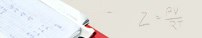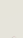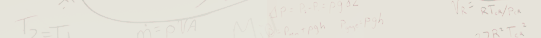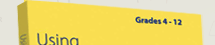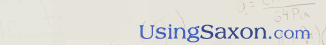Review the BookTable of ContentsJohn Saxon's LegacyYou Chose the Right BooksUse of CalculatorsGeometry CreditAbout the Author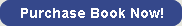As John Saxon observed during his world travels, the United States is the only industrialized nation in the world that neatly packages its mathematics into separately titled courses like Algebra, Geometry, Trigonometry, etc. If you were to meet international students coming to the United States as high school exchange students, you would notice they arrive with a single high school math textbook that contains algebra, geometry, trigonometry and calculus. John Saxon wanted to integrate geometry concepts throughout his math books instead of isolating them in individual chapters or books as other publishing companies were doing. Interwoven in all of John Saxon's math books is introductory, basic, and advanced geometry depending upon the level of the book. The actual geometry terms start as far back as Math 54 in the hardback textbooks. But it is not until students reach Algebra 2 that they encounter concepts normally found in high school geometry textbooks. The question then arises, “Is this formal or informal geometry?” What that question is really asking is whether or not the students do formal two-column proofs. Without the formal two-column proofs, the old high school geometry teachers referred to the textbook material as Informal Geometry . With the formal two-column proofs, it was described as Formal Geometry. In John Saxon's math books, students who complete the entirety of the second or third edition of Algebra 2 have also completed the equivalent of the first semester of Formal Geometry . If parents would rather use another term, Analytic Geometry could be used. While finishing the first half of Advanced Mathematics, the student completes the equivalent of the second semester of formal or Analytic Geometry . Students continue to do geometry problems and proofs throughout the remainder of the textbook, but no new geometry concepts are introduced. Whenever I am asked what geometry title to put on the transcript, I tell parents to use just the title of Geometry. I cannot recall ever seeing a high school transcript that recorded Formal Geometry, Informal Geometry, Analytic Geometry, non-Analytic Geometry, Euclidean Geometry, or non-Euclidean Geometry. The transcript reflected just Geometry. The advent of computer programming courses in high school has created a unique situation where the same skills encountered doing two-column geometry proofs can now be learned by taking a computer programming course. The geometry learned through the first half of Advanced Mathematics is all that is needed to be successful in that area on the ACT or SAT tests. I see no reason to add another separate geometry course. However, if parents desire that students take a separate geometry course, they should not place that course between Algebra 1 and Algebra 2 . During the time lapse, which could be as long as twelve to fifteen months, the average student will have forgotten many of the concepts encountered in Algebra 1 necessary for success in Algebra 2. Home | Review the book | Purchase book | Testimonials | Newsletter | Contact Us © 2006 - 2019 AJ Publishers, LLC